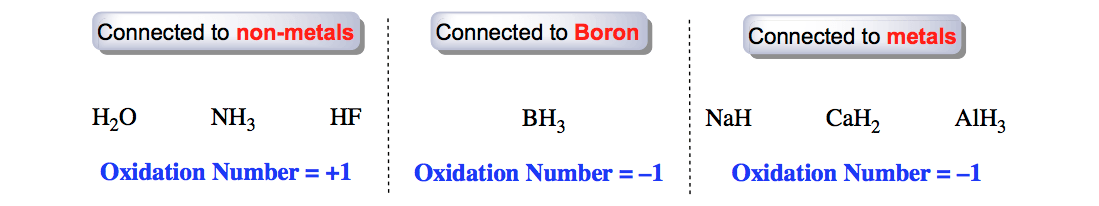Clutch Prep is now a part of Pearson
Ch.4 - Chemical Quantities & Aqueous ReactionsWorksheetSee all chapters

# Oxidation Number (IGNORE)

See all sections
Sections
Solutions
Molarity
Osmolarity
Dilutions
Solubility Rules
Electrolytes
Molecular Equations
Gas Evolution Equations
Solution Stoichiometry
Complete Ionic Equations
Calculate Oxidation Numbers
Redox Reactions
Balancing Redox Reactions: Acidic Solutions
Balancing Redox Reactions: Basic Solutions
Activity SeriesJules Bruno

The oxidation number, also known as oxidation state, represents the number of electrons that an element can either gain, lose or share to form a chemical bond with another element.

Oxidation Number

By definition, the oxidation number can be seen as the potential charge an atom can possess from either the sharing, gaining or loss of electrons in an oxidation-reduction reaction.

Calculating Oxidation Number

In order to calculate the oxidation of an element or compound we can utilize the following rules.

Rule 1: For an atom in its standard or elemental state its oxidation number is equal to zero. The following elements and molecules are good examples.

C (graphite)              O2 (g)              P4 (s)              Cl­2 (g)             He (g)             Zn (s)

Rule 2: For an ion, its charge is equal to its oxidation number.

Na+  Oxidation Number = +1                     SO42–  Oxidation Number = – 2

Rule 3: For metals from Group 1A, their oxidation number is equal to +1 when connected to other elements.Oxidation Number (Group 1A)

Rule 4: For metals from Group 2A, their oxidation number is equal to +2 when connected to other elements.Oxidation Numbers (Group 2A)

Rule 5: For hydrogen, the oxidation number is +1 when connected to non-metals and -1 when connected to boron or a metal.Oxidation Numbers (Hydrogen)

Rule 6: For fluorine, the oxidation number is -1 when connected to other elements.Oxidation Number (Fluorine)

Rule 7: For oxygen, the oxidation number is -1 when it’s a peroxide, -1/2 when it’s a superoxide and -2 in most other cases.

Peroxide

A peroxide represents a compound containing two elements from Group 1A bonded to two oxygen atoms.Oxidation Number (Peroxides)

Superoxide

A superoxide represents a compound containing one element from Group 1A bonded to two oxygen atoms.Oxidation Number (Superoxides)

Rule 8: For the halogens (Cl, Br, I), their oxidation numbers are -1 unless they are connected to oxygen.Oxidation Number (Halogens)

When the halogen (Cl, Br, I) is connected to oxygen then the oxidation number must be calculated.Calculating Oxidation Number (Perbromate Ion)

Now let’s take a look at a few practice problems.

PRACTICE 1: Determine the oxidation number of the sulfur atom in the sulfate ion.Calculating Oxidation State (Sulfate Ion)

PRACTICE 2: Determine the oxidation number of the nitrogen atom.Calculating Oxidation Number (Ammonium Ion)

PRACTICE 3: Determine the oxidation number of the carbon atom in carbon dioxide.Calculating Oxidation Number (Carbon dioxide)

Beyond Oxidation Numbers

Oxidation Numbers can serve roles beyond redox reactions such as naming ionic compounds, determining the degree of oxidation within biological reactions, understanding electron configurations and other trends of the periodic table.Jules Bruno

Jules felt a void in his life after his English degree from Duke, so he started tutoring in 2007 and got a B.S. in Chemistry from FIU. He’s exceptionally skilled at making concepts dead simple and helping students in covalent bonds of knowledge.